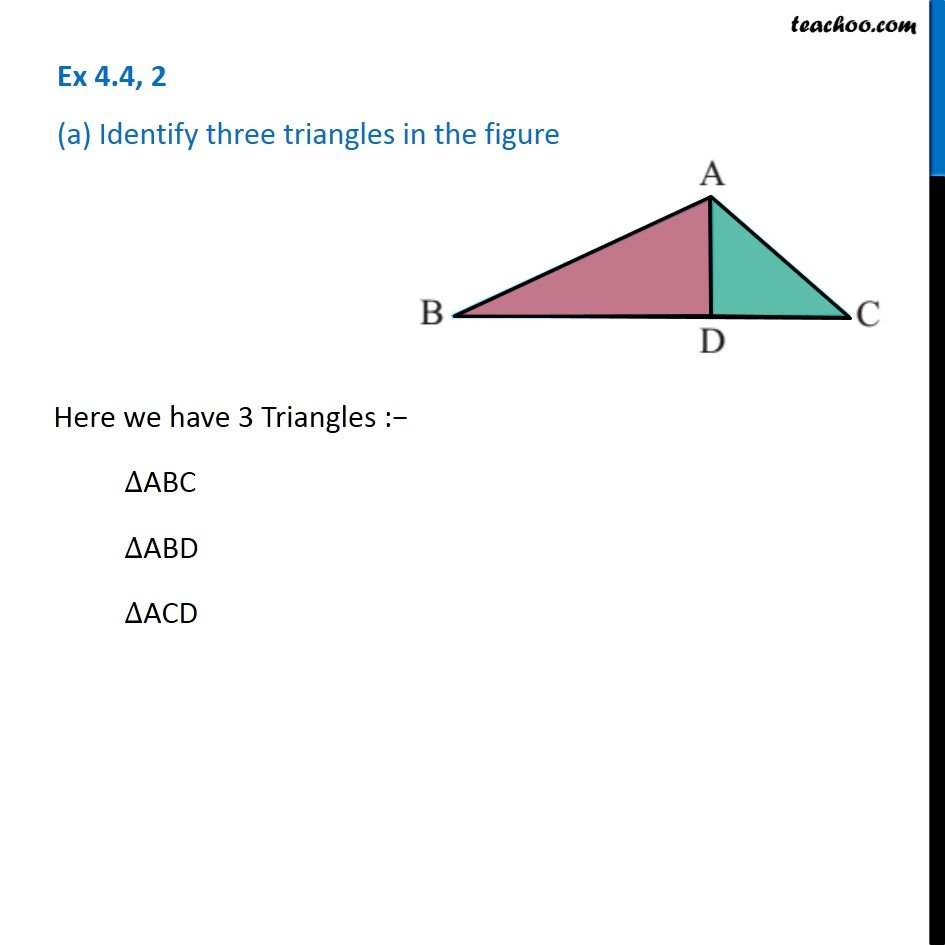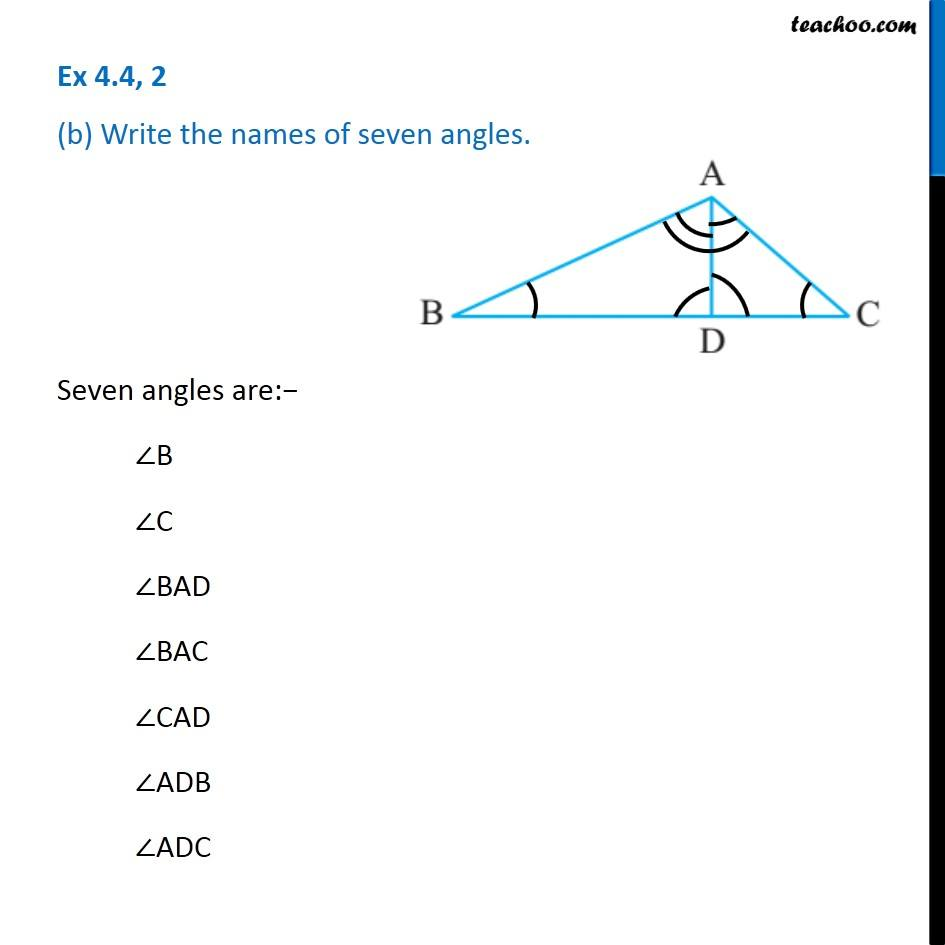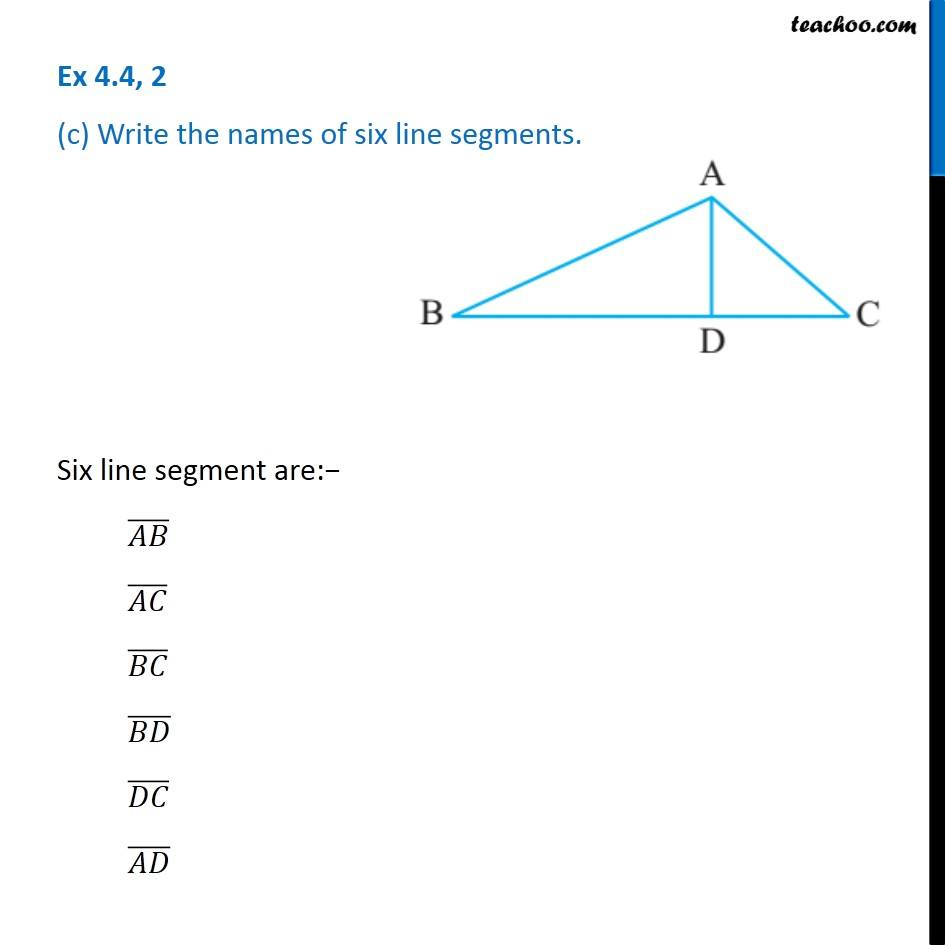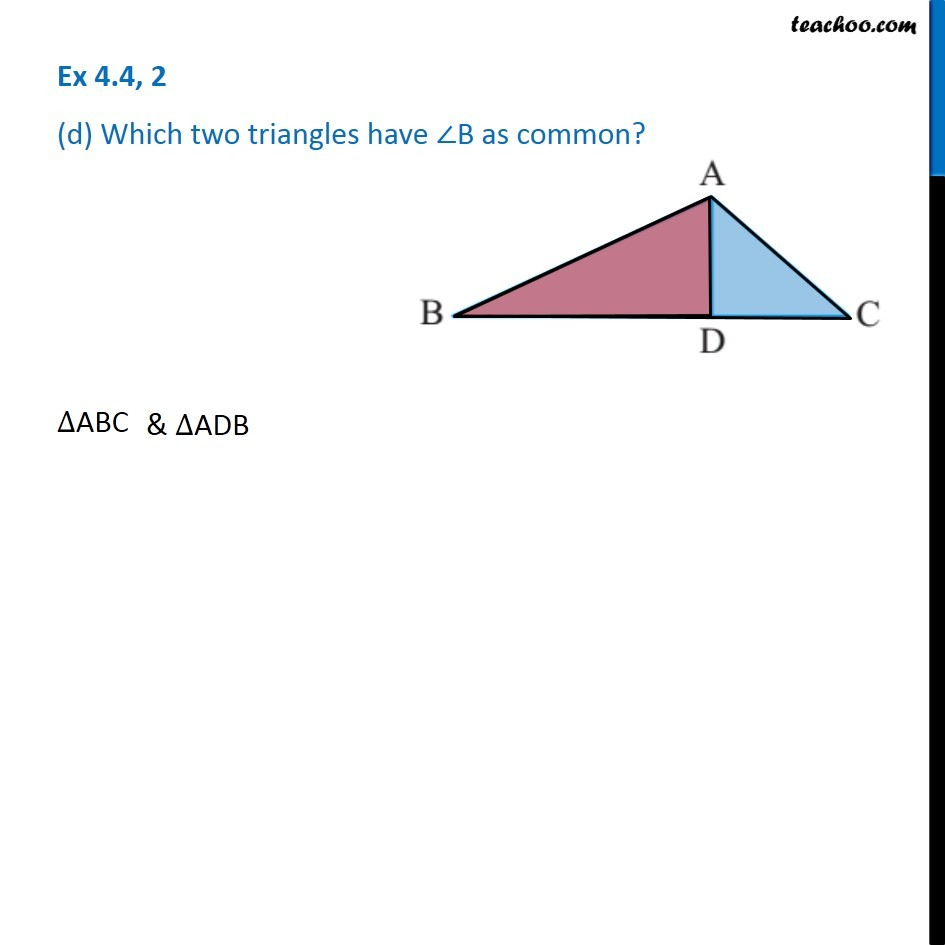Triangles

Chapter 4 Class 6 Basic Geometrical Ideas
Serial order wiseLearn in your speed, with individual attention - Teachoo Maths 1-on-1 Class

### Transcript

Question 2 (a) Identify three triangles in the figure Here we have 3 Triangles :− ∆ABC ∆ABD ∆ACD Question 2 (b) Write the names of seven angles. Seven angles are:− ∠B ∠C ∠BAD ∠BAC ∠CAD ∠ADB ∠ADC Question 2 (c) Write the names of six line segments. Six line segment are:− (𝐴𝐵) ̅ (𝐴𝐶) ̅ (𝐵𝐶) ̅ (𝐵𝐷) ̅ (𝐷𝐶) ̅ (𝐴𝐷) ̅ Question 2 (d) Which two triangles have ∠B as common? ∆ABC